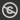Science, Maths & Technology
Free course

# Dynamics## Course reviews

This free course is concerned with moving objects, that is, dynamics. Section 1 introduces concepts like position, velocity and acceleration, which describe the way an object moves. Section 2 discusses Newton’s laws of motion, which predict the motion of an object when the forces acting on it are known. Section 3 shows how Newton’s second law of motion can be used to predict the motion of objects. Section 4 concerns modelling some of the forces that occur in nature, which enables more realistic situations to be analysed. Section 5 looks at motion in more than one dimension.

## Course learning outcomes

After studying this course, you should be able to:

• understand and use basic terms for the description of the motion of particles, vector functions and the fundamental laws of Newtonian mechanics
• solve mechanics problems in one dimension that involve one or more of the forces of gravity, friction and air resistance
• understand the concept of terminal speed, and use it in solving mechanics problems in one dimension
• apply Newton's second law in vector form to problems in more than one dimension
• solve problems relating to the motion of a projectile in the absence of air resistance.

First Published: 13/12/2017

Updated: 22/07/2019

Skip Rate and Review